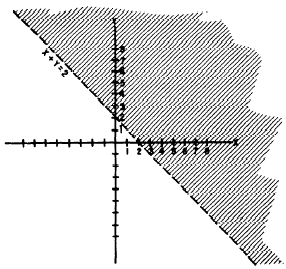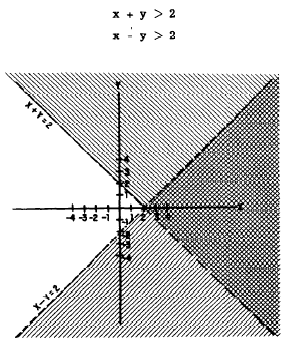Plotting on the coordinate systemCustom SearchPLOTTING ON THE COORDINATE SYSTEM It would be extremely laborious to plot enough points at random to define an entire area of the coordinate system. Therefore our method consists of plotting a boundary line and shading the area, on one side of this line, wherein the solution points lie. The equation of the boundary line is formed by changing the inequation to an equation. For example, the equation of the boundary line for the graph of         x+y>2 is the equation         x+y=2 Figure 12-9 is a graph of x + y > 2. Notice that the boundary line x + y = 2 is not solid. This is intended to indicate that points on the boundary line are not members of the solution set. Every point lying above and to the right of the boundary line is a member of the solution set. Any solution point may be verified by substituting its X and Y coordinates for x and y in the original inequation.Figure 12-9.-Graph of x + y > 2. SIMULTANEOUS INEQUALITIES The areas representing the solutions of two different inequations may overlap. If such an overlap occurs, the. area of the overlap includes all points whose coordinates satisfy both inequations simultaneously. An example of this is shown in figure 12-10, in which the following two inequations are graphed:Figure 12-10.-Graph of x + y > 2 and x - y > 2. The double crosshatched area in figure 12-10 contains all points which comprise the solution set for the system.Integrated Publishing, Inc. - A (SDVOSB) Service Disabled Veteran Owned Small Business